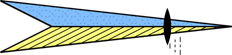RST Engineering

Kit Electronics For Aviation and CommunicationJim's Engineering Page

Adapt a 3¼" to a 2½" Instrument Hole  (pdf)

Variations in the atmosphere with altitude (Excel)

Dimensions for a 2½" Instrument Hole (pdf)

Dimensions for a 3¼" Instrument Hole (pdf)

SAE Machine Screw table including drill holes (pdf)

Dimensions for Inductors and Capacitors (Excel)

Ohm's Law Calculator (Excel)

Convert rectangular to polar dimensions (Excel)

RF Wavelengths and Ranges (Excel)

Calculations for a Sine Wave Oscillator (Excel)

Wire Tables and calculations (Excel)